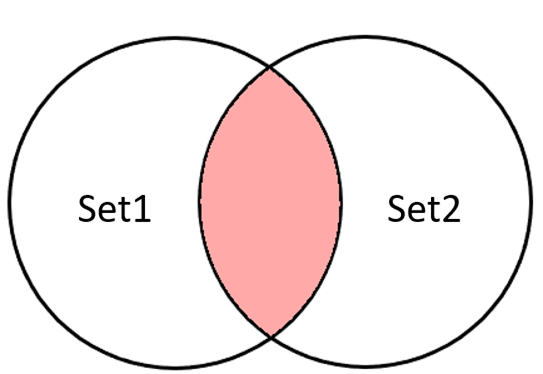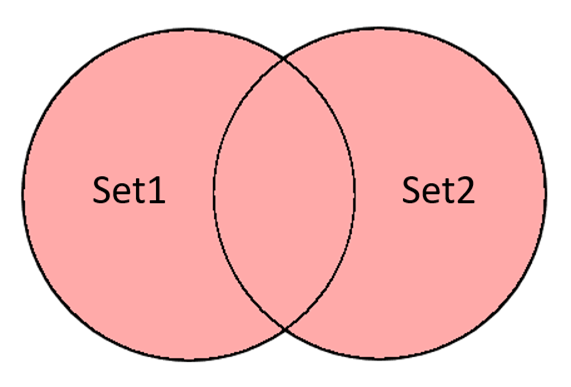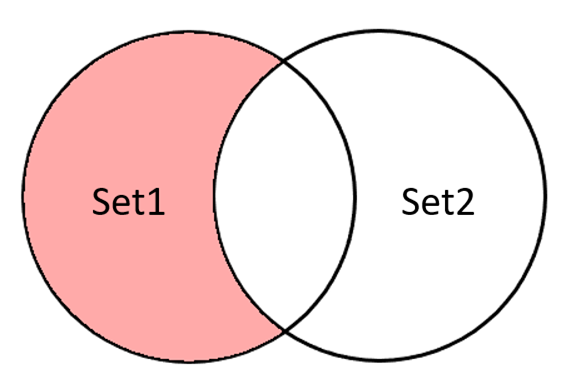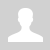Python. Sets (Множество)

Python. Set (Множество)

A set is a complex data type that represents several values (elements of a set) under one name.

A set is an unordered collection of unique items.

You can define a set by listing the elements of the set in curly braces.

For example,

```color_set = {"red", "yellow", "green"} print(color_set)```

Run this program several times. We can get outputting:

{"red", "yellow", "green"}

{"yellow",  "red", "green"}

{"red", "green", "yellow"}

and other different cases.

set is an unordered collection.

Sets

• does not contain identical elements;
• the order of the elements in the set is not defined;
• you can add and remove items;
• elements of sets can be of different types;
• you can iterate over the elements of the set;
• you can check whether an element belongs to a set;
• you can perform operations on sets (union, intersection, difference).

In a program, you can declare an empty and non-empty set as follows:

``````fruits = set () # empty set fruits
fruits = {"apple", "orange", "lemon", "banana"} # non-empty set of 4 elements
print(fruits) # output the set to the screen``````

Run this program several times. Watch the output. What have you noticed?

Indeed, the set of elements in the output is the same, but the order of output can change (the order of elements in the set is not defined), i.e. each time the program is started, its sequence of elements is generated.

Interesting! If you use the output of the same set multiple times in the same program, then the order in all outputs will be the same.

When displaying an empty set, we get on the screen: set ()

``````fruits = set() # empty set fruits
print(fruits) # will print set()``````

The elements of a set can have different types.

``````# set fruits_and_price contains both string and integer elements
fruits_and_price = {"apple", 300, "orange", 700, "lemon", 900, "banana", 550}``````

The set only stores unique elements, i.e. those that do not repeat others.

For example, if we write repeating elements in a set, the set will only store one element.

``````# the fruits set contains two "orange" elements
fruits = {"apple", "orange", "lemon", "orange", "banana"}

# only 4 non-repeating elements will be printed
print(fruits)``````

### Set functions len(), sum(), min(), max()

``````numbers = {5, 3, 7, 4}
print(len(numbers)) # length of set fruits = 4
print(sum(numbers)) # the sum of the elements of the set (only for numbers) = 19
print(min(numbers)) # minimum value of the set = 3
print(max(numbers)) # maximum value of the set = 7
``````

### Set operations

1. Calculation of the number of elements in a set

len(set_variable)

``````fruits = {"apple", "orange", "lemon", "banana"}
print(len(fruits)) # length of fruits set = 4

fruits_and_price = {"apple", 300, "orange", 700, "lemon", 900, "banana", 550}
print(len(fruits_and_price)) # length of set fruits_and_price = 8``````

2. Looping through the elements of the set

for item in my_set:

``````fruits = {"apple", "orange", "lemon", "banana"}
for item in fruits: # iterate over the elements of the set
print(item) # output of a set item, the order of output may differ``````

3. Search for an item in a set

if item in my_set:

``````if item in my_set: # check if items are in the set
print('The item is in the set')
else:
print('The item is not in set')``````

4. Adding an element to the set

``````fruits = {"apple", "orange", "lemon", "banana"}
fruit = "melon"
print(len(fruits)) # the number of items will be 5
``````

If we add an element that is already there, then the set of elements of the set will not change.

``````my_set = set() # declare an empty set
print(my_set) # will print a set of two elements {1, 5}``````

5. Deleting an item

Three different methods are used to remove an element from a set:

discard - removes the given element, if it is in the set, and does nothing if it is not;

``````my_set = {'one', 'two', 'three'}
my_set.discard('two') # element 'two' removed from set my_set
my_set.discard('four') # item not deleted, no error
print(my_set) # will print the remaining elements of the set {'one', 'three'}
``````

remove  - removes the given element, if any, and throws a KeyError if not;

``````my_set = {'one', 'two', 'three'}
my_set.remove('two') # element 'two' removed from my_set
print(my_set) # will print the remaining elements of the set {'one', 'three'}
my_set.remove('four') # cannot be removed, KeyError``````

The remove method will allow you to check for an error in the program. If you are sure that an element should be in a set, it is better to get an error during debugging and fix it right away than to spend time searching if the program is not working properly.

pop - removes some element from the set and returns it as a result. In this case, the order of deletion is unknown.

``````my_set = {'one', 'two', 'three'}
print('before deletion:', my_set)
item = my_set.pop()
print('deleted item:', item)
print('after deletion:', my_set)``````

6.Clear set

my_set.clear ()

``````my_set = {'one', 'two', 'three'}
my_set.clear()
print(my_set)  # will print an empty set set()``````

### Operations on two sets

A new set can be obtained from two sets using the following operations:

 Operation Usage example Intersection```set1 = {5, 8, 4, 6} set2 = {1, 2, 8, 5} new_set = set1 & set2 print(new_set) new_set = set1.intersection(set2) print(new_set)```Result: {5, 8} Union```set1 = {5, 8, 4, 6} set2 = {1, 2, 8, 5} new_set = set1 | set2 print(new_set) new_set = set1.union(set2) print(new_set)```Result: {1, 2, 4, 5, 6, 8} Difference```set1 = {5, 8, 4, 6} set2 = {1, 2, 8, 5} new_set = set1 - set2 print(new_set) new_set = set1.difference(set2) print(new_set)```Result: {4, 6} Symmetric Difference```set1 = {5, 8, 4, 6} set2 = {1, 2, 8, 5} new_set = set1 ^ set2 print(new_set) new_set = set1.symmetric_difference(set2) print(new_set)```   Result: {1, 2, 4, 6}

## Comparison of sets

``````print({1, 2, 3, 4} == {4, 3, 1, 2}) # True
print({1, 3, 2, 4} != {4, 3, 1, 2}) # False
print({"one", "two", "three"} >= {"one", "three"}) # True
print({2, 4} < {1, 3, 4}) # False
print({"one", "more", "time"} < {"time", "one", "more"}) # False``````

Subset and superset

``````s1 = {'a', 'b', 'c'}
print(s1 <= s1) # True
s2 = {'a', 'b'}
print(s2 <= s1) # True
s3 = {'a'}
print(s3 <= s1) # True
s4 = {'a', 'z'}
print(s4 <= s1) # False``````

The operation s1 < s2 means "s1 is a subset of s2 but does not completely coincide with it" i.e. all elements of s1 are in s2.

The operation s1 > s2 means "s1 is a superset of s2, but does not completely coincide with it", i.e. s1 elements contain all s2 elements.

Questions:

1. What is a set in Python programming?
2. Describe features of the set.

Exercises:

Ex. 1 "Calculate results"

Ex. 2 "Compare sets"

Ex. 3 "Set operations"

Ex. 4 "Results of set operations"

Категория: Algorithms | Добавил: bzfar77 (28.10.2021)
Просмотров: 772 | Теги: Set, Python, data structure, data type | Рейтинг: 5.0/3
 Всего комментариев: 0